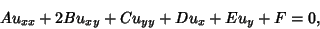## Hyperbolic Partial Differential Equation

A Partial Differential Equation of second-order, i.e., one of the form(1)

is called hyperbolic if the Matrix(2)

satisfies det. The Wave Equation is an example of a hyperbolic partial differential equation. Initial-boundary conditions are used to give(3)(4)(5)

where(6)

holds in.

See also Elliptic Partial Differential Equation, Parabolic Partial Differential Equation, Partial Differential Equation